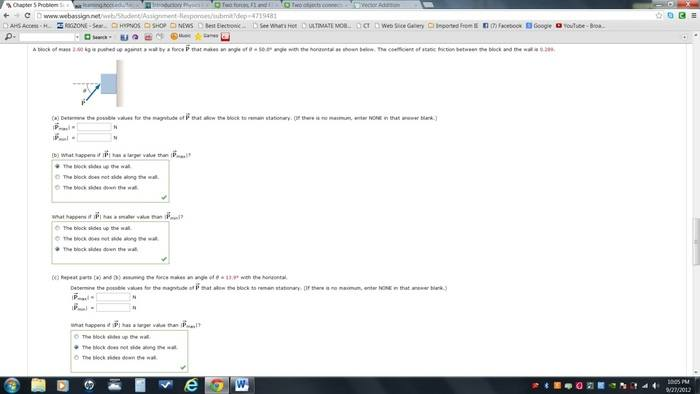# Finding the possible values of a force pushing a block

AryRezvani

## Homework Statement## Homework Equations

I believe the only necessary equations would be those utilized to find the X and Y components of a certain force as well as the formula used to find μs

µ = ffriction/fnormal

## The Attempt at a Solution

Attempt:

I'm not really sure where to start. If someone could just give me a push in the right direction, it'd be much appreciated.Homework Helper

## Homework Statement## Homework Equations

I believe the only necessary equations would be those utilized to find the X and Y components of a certain force as well as the formula used to find μs

µ = ffriction/fnormal

## The Attempt at a Solution

Attempt:

I'm not really sure where to start. If someone could just give me a push in the right direction, it'd be much appreciated.In all cases, the Normal Reaction Force [to be used in the Friction = μN] is the horizontal component of the applied Force, which they called P.

Also in all cases, the vertical forces acting on the block are: mg, down, Pvert, up plus friction.

If you push with too small a force, the block will slip down, so friction will act up. When the force becomes big enough to stop the slip, Pvert, up + friction, up will equal mg, down.

If you push too hard, the block will slip up, so friction will act down. At the point of slipping

Pvert, up will equal mg, down plus friction, down

Note: Pvert, up means the vertical component of the applied force P